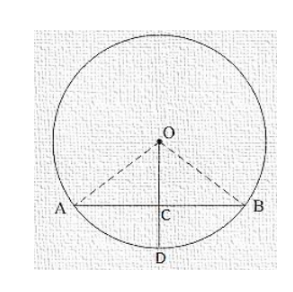# Prove that the line joining the mid-point of a chord to the centre of the circle passes through the mid-point of the

Question:

Prove that the line joining the mid-point of a chord to the centre of the circle passes through the mid-point of the corresponding minor arc.

Solution:

Given:

C is the mid-point of chord AB.

To prove: D is the mid-point of arc AB.

Proof:

In ΔOAC and ΔOBC

OA = OB [Radius of circle]

OC = OC [Common]

AC = BC [C is the mid-point of AB]

Then ΔOAC ≅ ΔOBC [By SSS condition]

∴ ∠AOC = ∠BOC$\Rightarrow \mathrm{m} \overline{\mathrm{A}} \mathrm{D} \cong \mathrm{m} \overline{\mathrm{B}} \mathrm{D}$

$\Rightarrow \overline{\mathrm{A}} \mathrm{D} \cong \overline{\mathrm{B}} \mathrm{D}$

Hence, D is the mid-point of arc AB.